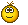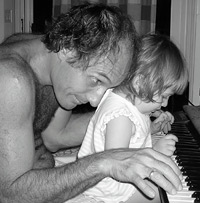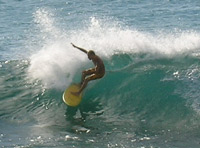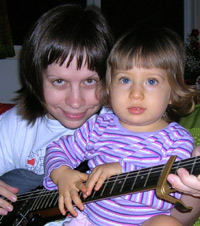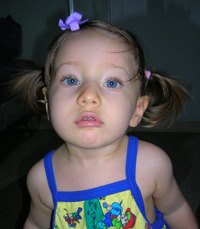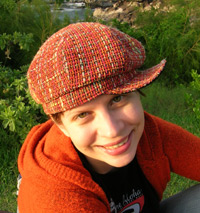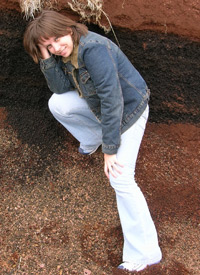P h o t o s  o n  t h i s  p a g e:

 G a r y  &  M a s h a  a r e  p l a y i n g  s y n t h e s i z e r
 G a r y  i s  s u r f i n g  'H o l e  i n  t h e  H e a d'
 M a s h a  w i t h  M i l l a  J o v o v i c h  e x p r e s s i o n
 I r i n a  &  h e r  'b e l o v e d'  h a t  [i f  i t  o n l y  w a s  p o s s i b l e  t o  s l e e p  w e a r i n g  i t !]
 M a s h a , M a m a , &  P a p a ' s  g u i t a r  ^
 I r i n a  &  r e d  d i r t  a t  H a l e a k a l a  >